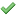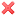# Thread: comparing graphs of functions

1. ## comparing graphs of functions

Compare the functions f(x) = x7 and g(x) = 7x by graphing both functions in several viewing rectangles. (a) Find all points of intersection of the graphs correct to one decimal place.
( 1, 2) (smaller x value)
( 3, 4) (larger x value)

I cant seem to find the points when i put it in my calculator. any help?

2.Originally Posted by kyleu03Compare the functions f(x) = x7 and g(x) = 7x by graphing both functions in several viewing rectangles. (a) Find all points of intersection of the graphs correct to one decimal place.
( 1, 2) (smaller x value)
( 3, 4) (larger x value)

I cant seem to find the points when i put it in my calculator. any help?
what is the significance of the red 7 ?

is it an exponent in f(x) ?

is
$\displaystyle g(x) = 7x$ or $\displaystyle 7^x$ ?

use a caret (^) symbol to denote exponents ... i.e. x^7 is x to the 7th power.

3. it is just the number, it is red because its changes. idk how to do it

4.Originally Posted by kyleu03it is just the number, it is red because its changes. idk how to do it
This does not answer the questions raised and will just delay you getting a helpful reply. Re-post the equations. Use the conventional formatting for exponents (that is, powers).

Note: x^7 means $\displaystyle x^7$, 7^x means $\displaystyle 7^x$,

5.Originally Posted by kyleu03it is just the number, it is red because its changes. idk how to do it
x7 makes no sense. what is f(x) ?

6. ohh im very sorry i didnt see it posted wrong. it is

f(x) = x^7
g(x) = 7^x

7.Originally Posted by kyleu03ohh im very sorry i didnt see it posted wrong. it is

f(x) = x^7
g(x) = 7^x
one solution should be obvious ...

graph $\displaystyle y = x^7 - 7^x$ and look for the the other zero ... easier to find.

8. "graphand look for the the other zero " does not find the points of intersection for me

9.Originally Posted by kyleu03"graphand look for the the other zero " does not find the points of intersection for me
An obvious solution is a whole number that lies somewhere between 5 and 8 ....

The other solution solution cannot be found exactly. A decimal approximation lies somewhere between 1 and 2. You need to use your graph (or graphs) to estimate the value correct to 1 decimal place.

As to why you can't find the intersection points when you put it into your calculator, you should check:

1. that you've entered the correct equations.

2. you're using an appropriate window (check that ymin and ymax of the window are OK).

10. I was able to find the smaller, but not larger. any help?

Compare the functions f(x) = x7 and g(x) = 7x by graphing both functions in several viewing rectangles.
(a) Find all points of intersection of the graphs correct to one decimal place.
( 1, 2) (smaller x value)
( 3, 4) (larger x value)

(b) Which function grows more rapidly when x is large? 5
f(x)
g(x)

11.Originally Posted by mr fantasticAn obvious solution is a whole number that lies somewhere between 5 and 8 ....

The other solution solution cannot be found exactly. A decimal approximation lies somewhere between 1 and 2. You need to use your graph (or graphs) to estimate the value correct to 1 decimal place.

[snip]Originally Posted by kyleu03I was able to find the smaller, but not larger. any help?
[snip]
There aren't many whole numbers between 5 and 7 that require testing ....

12. are you saying (0,7)

13.Originally Posted by kyleu03are you saying (0,7)
I am saying that you should check what happens when x = 6 and x = 7.

14. thats wrong blong

15.Originally Posted by kyleu03thats wrong blong
For crying out loud! $\displaystyle f(x) = x^7$ and $\displaystyle g(x) = 7^x$ have an intersection point at x = 7. The value of each function at x = 7 is 7^7. Given the previous replies, this should not have needed spelling out.

comparing, functions, graphs